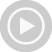Discover what you can learn.

With video courses for work, study and leisure.

# Algebra Tutor

By Jason Gibson

## Gain confidence in your abilities

The Algebra 2 Tutor is a 6 hour online course that will aid the student in the core topics of Algebra 2.

This online course bridges the gap between Algebra 1 and Trigonometry, and contains essential material to do well in advanced mathematics. Many of the topics in contained in this course are used in other Math courses, such as writing equations of lines, graphing equations, and solving systems of equations.

Learn with step-by-step example problems. Every topic in this course is taught by working problems so that the student gains confidence and skills immediately.

These skills are used time again in more advanced courses such as Physics and Calculus. Each topic is introduced by working example problems, beginning with the easier problems and gradually working the harder ones:

• Graphing Equations
• Writing Equations of Lines
• Solving Systems of Equations by Graphing and Substitution or Addition
• Simplifying Radical Expressions
• Multiplying and Dividing Radical Expressions
• Fractional Exponents

## ContentGraphing Equations 36:04The Slope of a Line 20:00Writing Equations of Lines 26:01Graphing inequalities 16:08Solving Systems of Equations by Graphing 28:28Solving Systems of Equations by Substitution 13:16Solving Systems of Equations by Addition 22:22Solving Systems of Equations in three Variables 18:15Simplifying Radical Expressions 18:26Adding and Subtracting Radical Expressions 23:17Multiplying and Dividing Radical Expressions 30:39Solving Equations with Radicals 19:42Fractional Exponents 26:52Solving Polynomial Equations 19:15The Quadratic Formula 10:09

## Details

• Included lectures: 15
• Duration: 5:28 h
• Available documents: 30

## Authors of course Algebra Tutor### Jason Gibson

Jason Gibson is the founder of MathTutorDVD.com and the teacher in all of the videos.

He also released DVDs in Physics and Basic Math and offers his courses online. His ongoing project is a comprehensive Chemistry series and an Engineering Circuit Analysis course.

### Customer reviews

(1)
5,0 of 5 stars
 5 Stars 5 4 Stars 0 3 Stars 0 2 Stars 0 1  Star 0